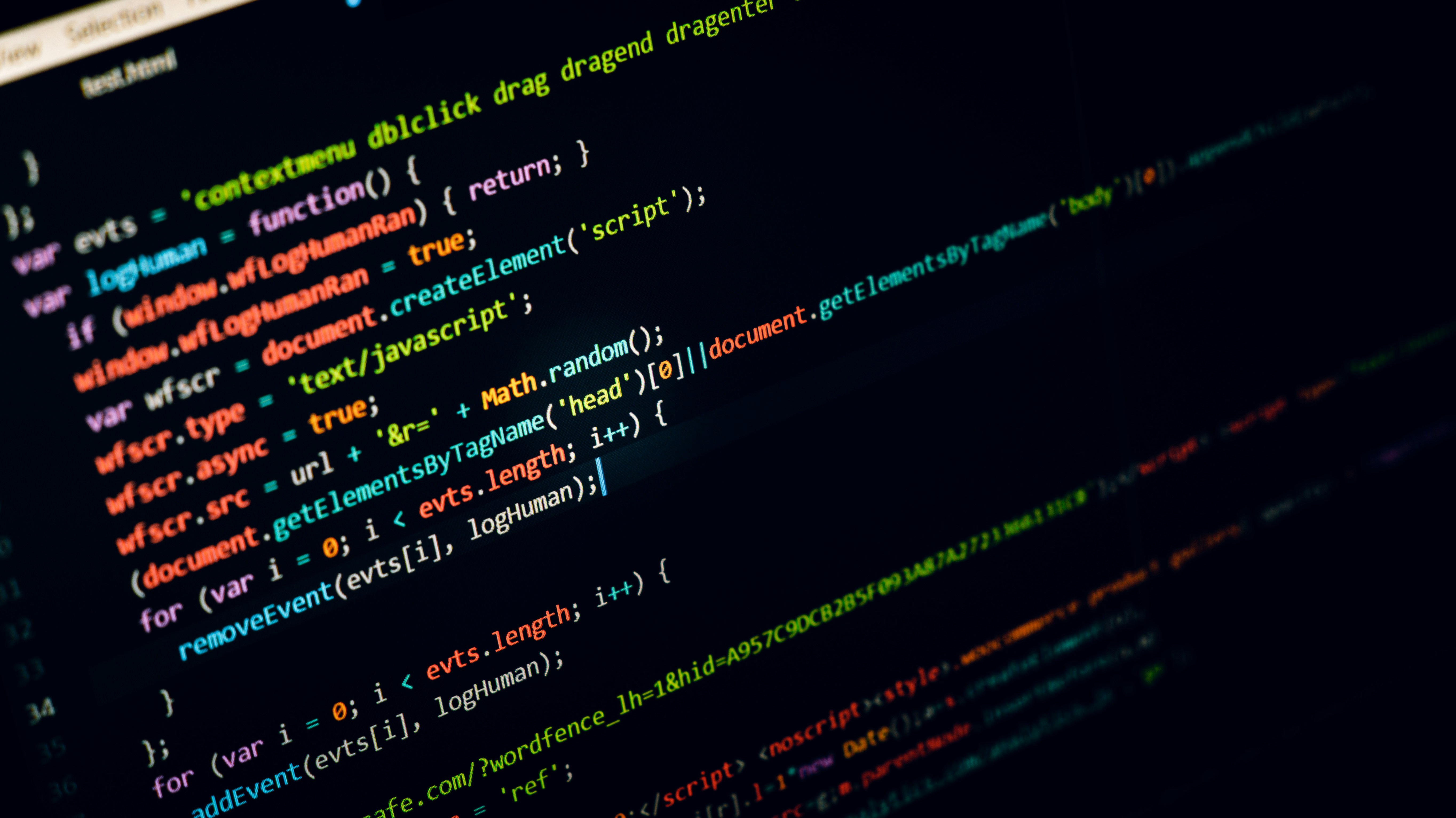# C++ 2. Escape SequencesIn part 1 you learned how to output a string to your screen. In this part, we will take a look at escape sequences. Escape sequences are basically characters that manipulate the string. When the backslash (\\), called an escape character, is followed by a special character, they form a escape sequence. The table below lists some common escape sequences.

 Escape sequence Description \n Newline \t Horizontal tab \r Carriage return \b Backspace \\ Backslash \' Single quote \" Double quote

I will show you source code for each one of the escape sequences followed by the output it produces quickly. The code I use when I go through the sequences here is:

``````#include <iostream>

int main() {
std::cout << "Hey you" << std::endl;
}``````

However, I will only show the relevant statement I'm changing. It's important that you have the main function if you want to try the code for yourself.

### \n

``	std::cout << "Hey \nyou" << std::endl;``
``````Hey
you``````

### \t

``	std::cout << "Hey \tyou" << std::endl;``
``Hey 	you``

### \r

``	std::cout << "Hey \ryou" << std::endl;``
``you``

### \b

``	std::cout << "Hey \byou" << std::endl;``
``Heyyou``

### \\

``	std::cout << "Hey \\you" << std::endl;``
``Hey \you``

### \'

``	std::cout << "Hey \'you" << std::endl;``
``Hey 'you``

### \"

``	std::cout << "Hey \"you" << std::endl;``
``Hey "you``